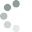# Schedule

## pdf version of the programme

Below you can browse the program filtering by different criteria.

Also you can use the search panel on the bottom of each page.

Legend:

 1. MLAC section Mathematical Logic, Algebra, and Combinatorics 2. GT section Geometry and Topology 3. A section Analysis 4. DEMP section Differential Equations and Mathematical Physics 5. AM section Applied Mathematics 6. MACS section Mathematical Aspects of Computer Science 7. MEHM section Mathematics Education and History of the Mathematics ms MMSC mini-symposium Mathematical Modelling and Scientific Computing ms NWI mini-symposium Nonlinear Waves and Integrability ms OCRT mini-symposium Optimal Codes and Related Topics ms T mini-symposium Topology